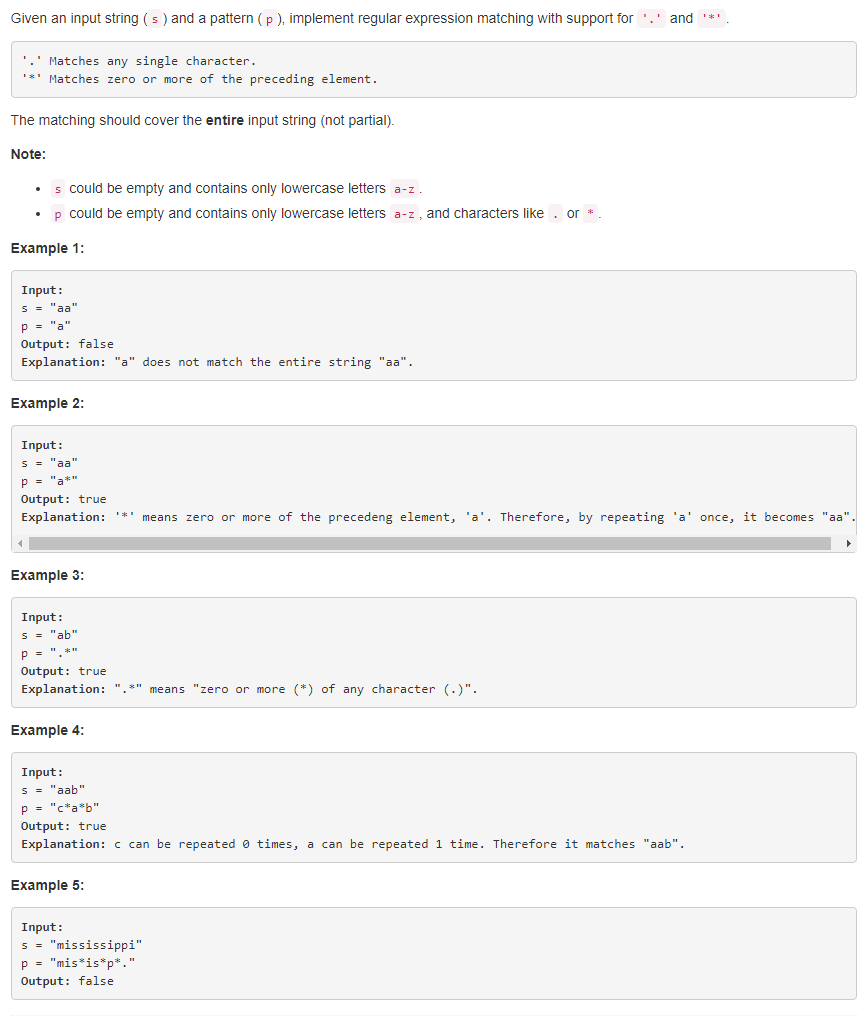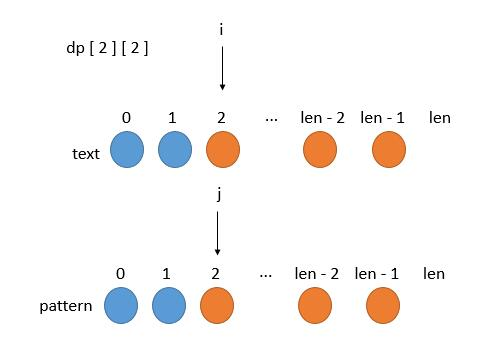# 题目描述（困难难度）# 解法一 递归

• 我们假设存在这么个函数 isMatch，它将告诉我们 text 和 pattern 是否匹配

boolean isMatch ( String text, String pattern ) ;

• 递归规模减小

text 和 pattern 匹配，等价于 text 和 patten 的第一个字符匹配并且剩下的字符也匹配，而判断剩下的字符是否匹配，我们就可以调用 isMatch 函数。也就是

(pattern.charAt(0) == text.charAt(0) || pattern.charAt(0) == '.')&&isMatch(text.substring(1), pattern.substring(1));

• 递归出口

随着规模的减小， 当 pattern 为空时，如果 text 也为空，就返回 True，不然的话就返回 False 。

if (pattern.isEmpty()) return text.isEmpty();


public boolean isMatch(String text, String pattern) {
if (pattern.isEmpty()) return text.isEmpty();

//判断 text 是否为空，防止越界，如果 text 为空， 表达式直接判为 false, text.charAt(0)就不会执行了
boolean first_match = (!text.isEmpty() &&
(pattern.charAt(0) == text.charAt(0) || pattern.charAt(0) == '.'));
return first_match && isMatch(text.substring(1), pattern.substring(1));
}


public boolean isMatch(String text, String pattern) {
if (pattern.isEmpty()) return text.isEmpty();

boolean first_match = (!text.isEmpty() &&
(pattern.charAt(0) == text.charAt(0) || pattern.charAt(0) == '.'));
//只有长度大于 2 的时候，才考虑 *
if (pattern.length() >= 2 && pattern.charAt(1) == '*'){
//两种情况
//pattern 直接跳过两个字符。表示 * 前边的字符出现 0 次
//pattern 不变，例如 text = aa ，pattern = a*，第一个 a 匹配，然后 text 的第二个 a 接着和 pattern 的第一个 a 进行匹配。表示 * 用前一个字符替代。
return (isMatch(text, pattern.substring(2)) ||
(first_match && isMatch(text.substring(1), pattern)));
} else {
return first_match && isMatch(text.substring(1), pattern.substring(1));
}
}


# 解法二 动态规划

...

text [ len，len ] 肯定好求

...

...$dp$就是图中橙色的部分.

public boolean isMatch(String text, String pattern) {
// 多一维的空间，因为求 dp[len - 1][j] 的时候需要知道 dp[len][j] 的情况，
// 多一维的话，就可以把 对 dp[len - 1][j] 也写进循环了
boolean[][] dp = new boolean[text.length() + 1][pattern.length() + 1];
// dp[len][len] 代表两个空串是否匹配了，"" 和 "" ，当然是 true 了。
dp[text.length()][pattern.length()] = true;

// 从 len 开始减少
for (int i = text.length(); i >= 0; i--) {
for (int j = pattern.length(); j >= 0; j--) {
// dp[text.length()][pattern.length()] 已经进行了初始化
if(i==text.length()&&j==pattern.length()) continue;

boolean first_match = (i < text.length() && j < pattern.length()
&& (pattern.charAt(j) == text.charAt(i) || pattern.charAt(j) == '.'));
if (j + 1 < pattern.length() && pattern.charAt(j + 1) == '*') {
dp[i][j] = dp[i][j + 2] || first_match && dp[i + 1][j];
} else {
dp[i][j] = first_match && dp[i + 1][j + 1];
}
}
}
return dp;
}


    public boolean isMatch(String text, String pattern) {
// 多一维的空间，因为求 dp[len - 1][j] 的时候需要知道 dp[len][j] 的情况，
// 多一维的话，就可以把 对 dp[len - 1][j] 也写进循环了
boolean[][] dp = new boolean[pattern.length() + 1];
dp[text.length()%2][pattern.length()] = true;

// 从 len 开始减少
for (int i = text.length(); i >= 0; i--) {
for (int j = pattern.length(); j >= 0; j--) {
if(i==text.length()&&j==pattern.length()) continue;
boolean first_match = (i < text.length() && j < pattern.length()
&& (pattern.charAt(j) == text.charAt(i) || pattern.charAt(j) == '.'));
if (j + 1 < pattern.length() && pattern.charAt(j + 1) == '*') {
dp[i%2][j] = dp[i%2][j + 2] || first_match && dp[(i + 1)%2][j];
} else {
dp[i%2][j] = first_match && dp[(i + 1)%2][j + 1];
}
}
}
return dp;
}